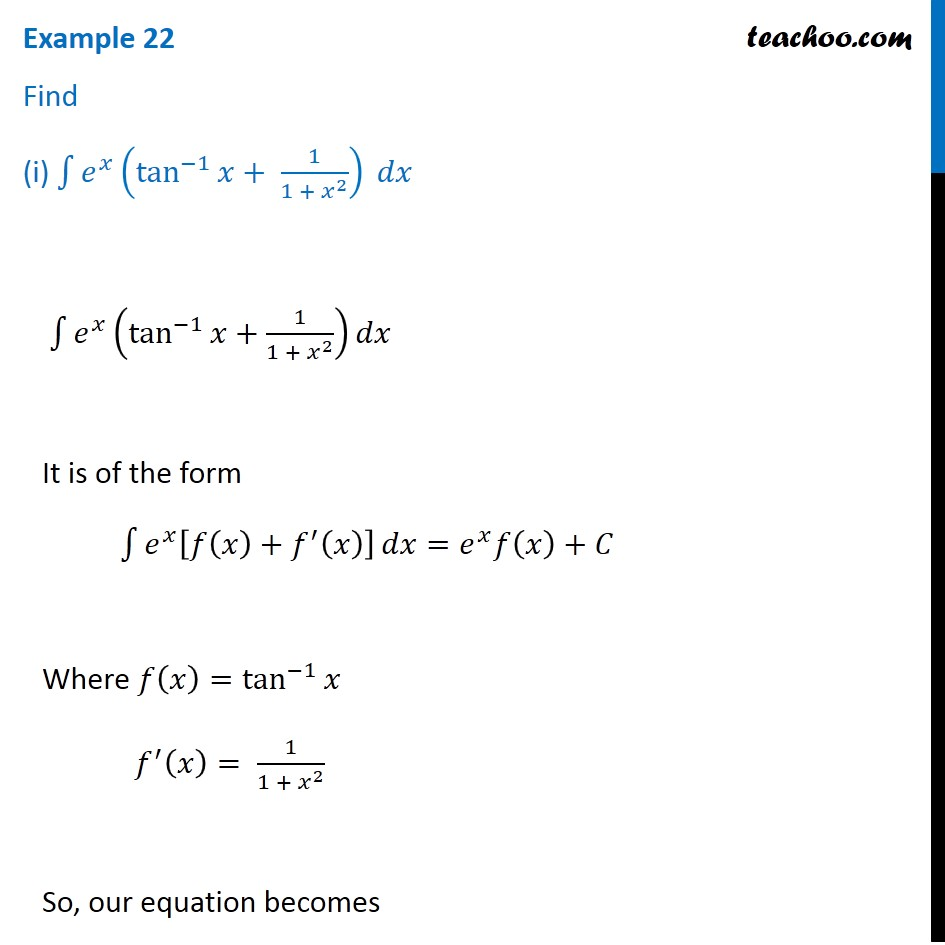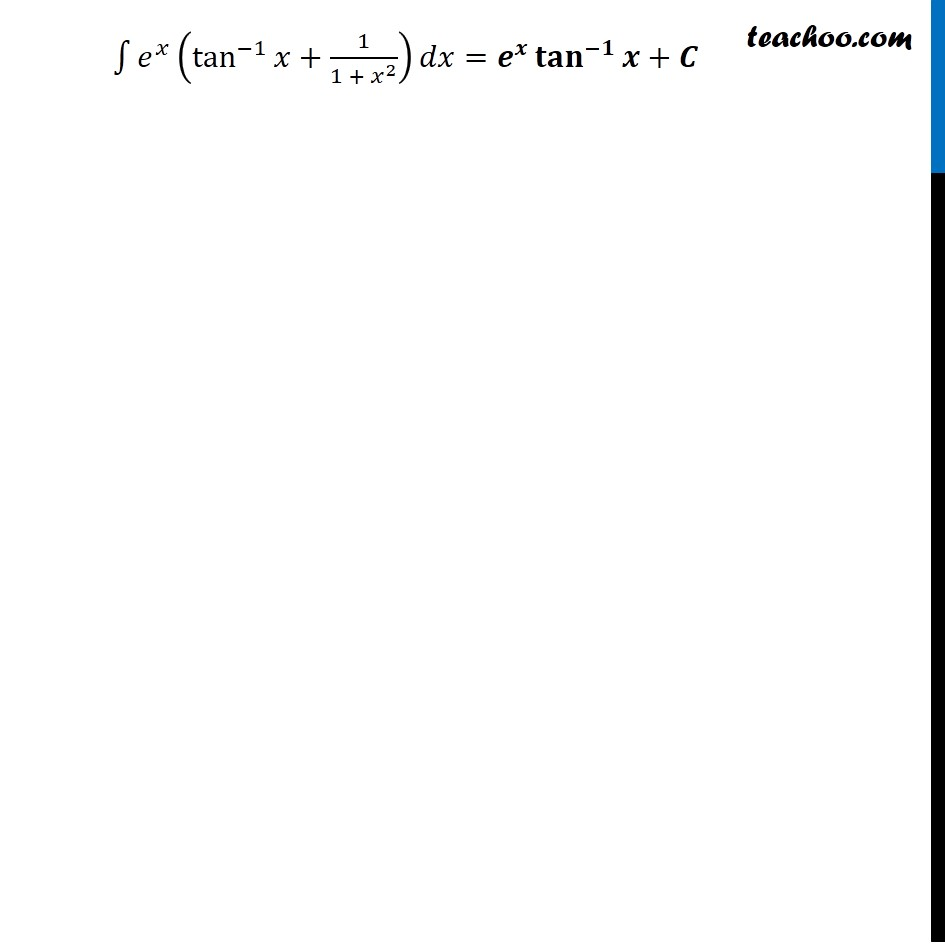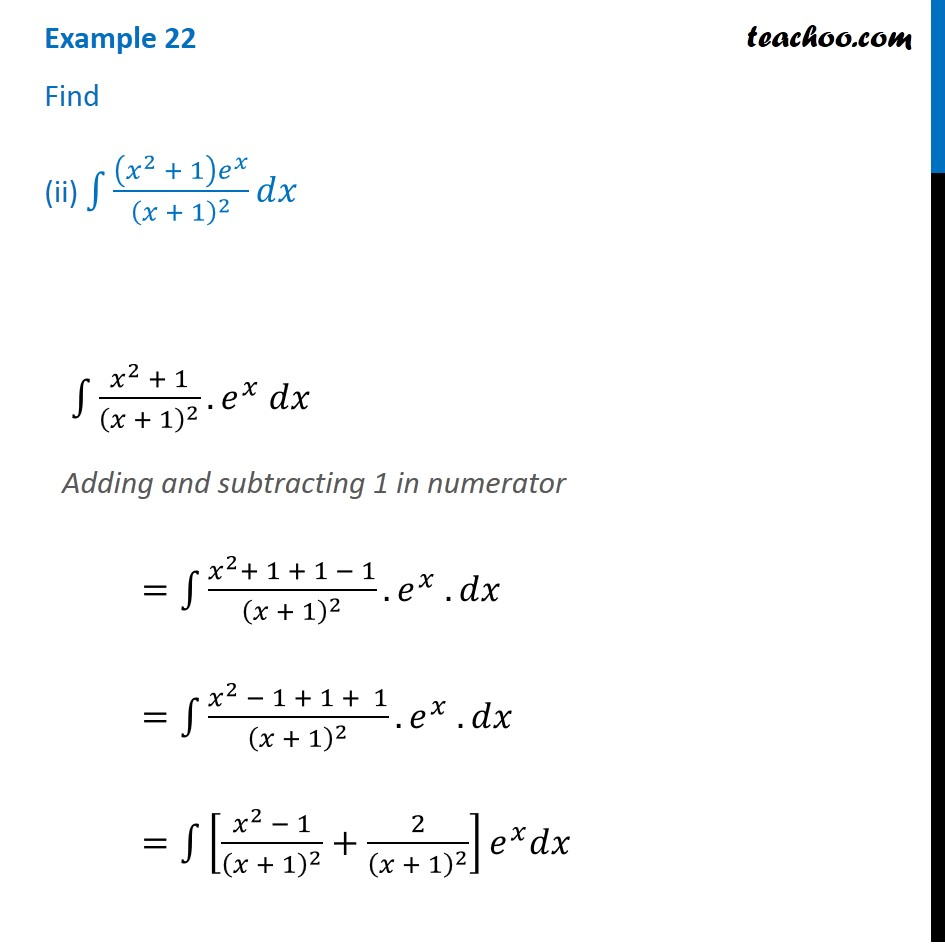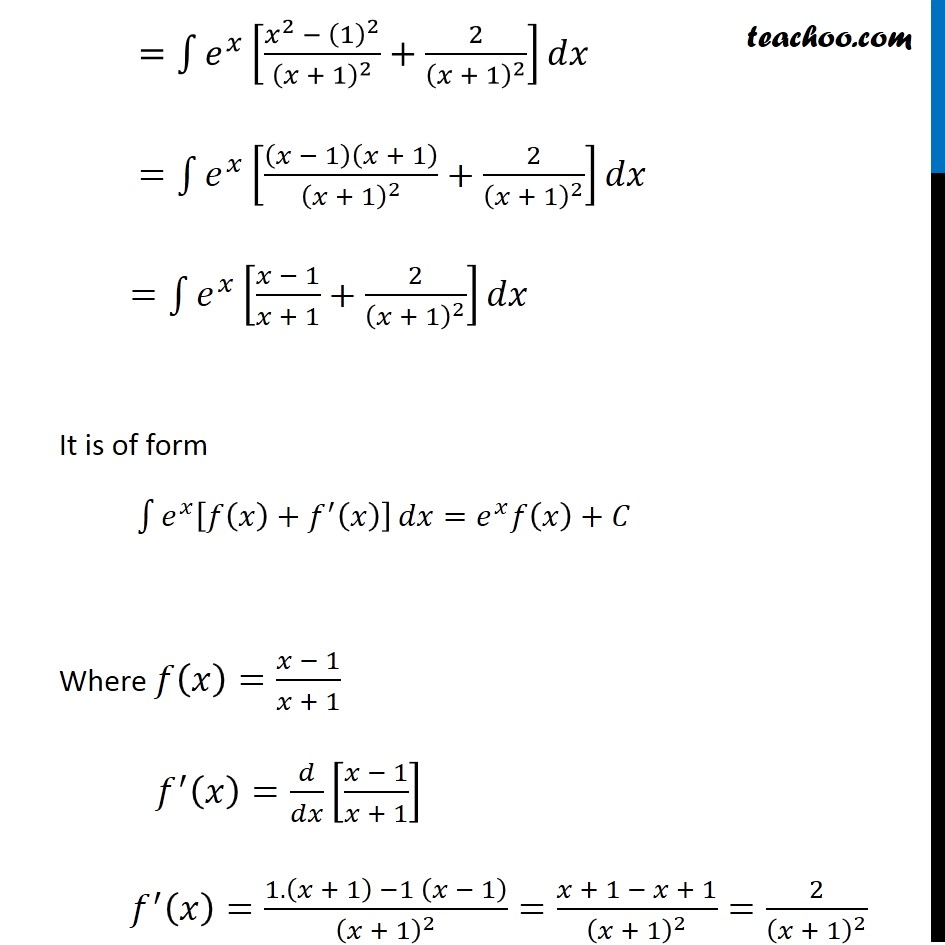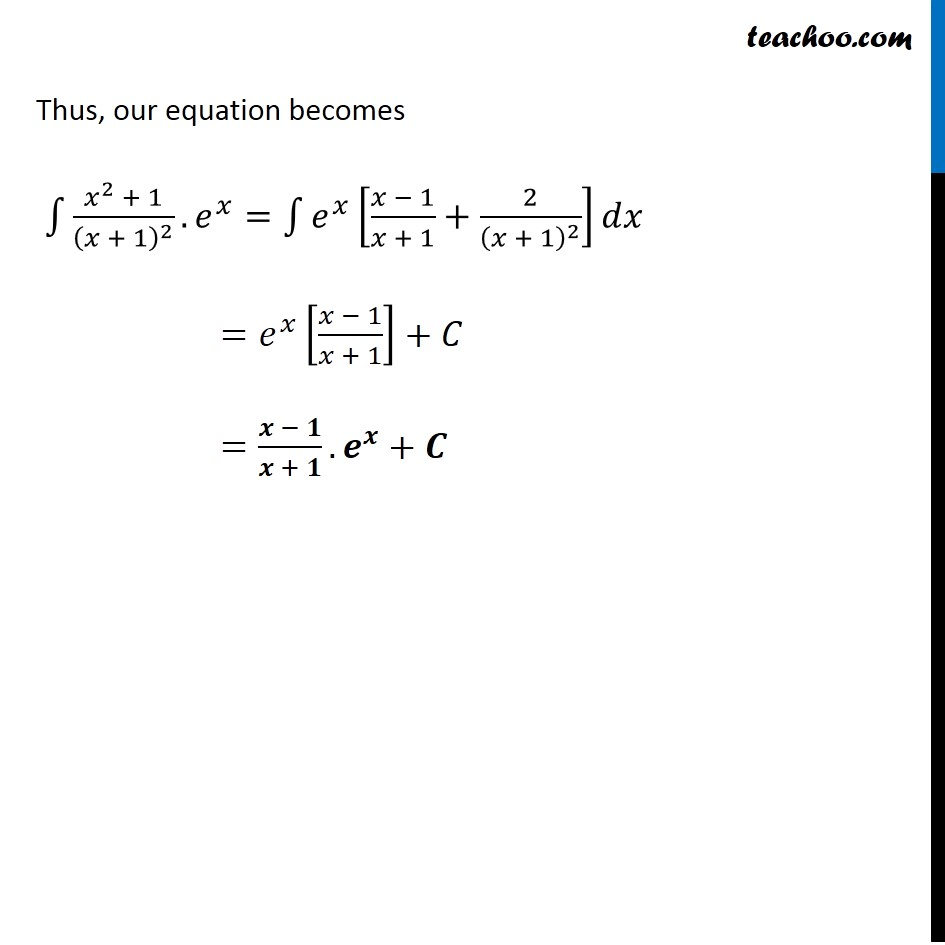1. Chapter 7 Class 12 Integrals (Term 2)
2. Concept wise
3. Integration by parts - e^x integration

Transcript

Example 22 Find (i) ∫1▒𝑒^𝑥 (tan^(−1)⁡𝑥+ 1/(1 + 𝑥^2 )) 𝑑𝑥 ∫1▒〖𝑒^𝑥 (tan^(−1)⁡𝑥+1/(1 + 𝑥^2 ))𝑑𝑥〗 It is of the form ∫1▒〖𝑒^𝑥 [𝑓(𝑥)+𝑓^′ (𝑥)] 〗 𝑑𝑥=𝑒^𝑥 𝑓(𝑥)+𝐶 Where 𝑓(𝑥)=tan^(−1)⁡𝑥 𝑓^′ (𝑥)= 1/(1 + 𝑥^2 ) So, our equation becomes ∫1▒〖𝑒^𝑥 (tan^(−1)⁡𝑥+1/(1 + 𝑥^2 ))𝑑𝑥〗=𝒆^𝒙 〖𝐭𝐚𝐧〗^(−𝟏)⁡〖𝒙+𝑪〗 Example 22 Find (ii) ∫1▒((𝑥^2 + 1) 𝑒^𝑥)/(𝑥 + 1)^2 𝑑𝑥 ∫1▒〖(𝑥^2 + 1)/(𝑥 + 1)^2 .𝑒^𝑥 𝑑𝑥〗 Adding and subtracting 1 in numerator =∫1▒〖(𝑥^2+ 1 + 1 − 1)/(𝑥 + 1)^2 .𝑒^𝑥 .𝑑𝑥〗 =∫1▒〖(𝑥^2 − 1 + 1 + 1)/(𝑥 + 1)^2 .𝑒^𝑥 .𝑑𝑥〗 =∫1▒〖[(𝑥^2 − 1)/(𝑥 + 1)^2 +2/(𝑥 + 1)^2 ] 𝑒^𝑥 𝑑𝑥〗 =∫1▒〖𝑒^𝑥 [(𝑥^2 − (1)^2)/(𝑥 + 1)^2 +2/(𝑥 + 1)^2 ]𝑑𝑥〗 =∫1▒〖𝑒^𝑥 [(𝑥 − 1)(𝑥 + 1)/(𝑥 + 1)^2 +2/(𝑥 + 1)^2 ]𝑑𝑥〗 =∫1▒〖𝑒^𝑥 [(𝑥 − 1)/(𝑥 + 1)+2/(𝑥 + 1)^2 ]𝑑𝑥〗 It is of form ∫1▒〖𝑒^𝑥 [𝑓(𝑥)+𝑓^′ (𝑥)] 〗 𝑑𝑥=𝑒^𝑥 𝑓(𝑥)+𝐶 Where 𝑓(𝑥)=(𝑥 − 1)/(𝑥 + 1) 𝑓^′ (𝑥)=𝑑/𝑑𝑥 [(𝑥 − 1)/(𝑥 + 1)] 𝑓^′ (𝑥)=(1.(𝑥 + 1) −1 (𝑥 − 1))/(𝑥 + 1)^2 =(𝑥 + 1 − 𝑥 + 1)/(𝑥 + 1)^2 =2/(𝑥 + 1)^2 Thus, our equation becomes ∫1▒〖(𝑥^2 + 1)/(𝑥 + 1)^2 .𝑒^𝑥=∫1▒〖𝑒^𝑥 [(𝑥 − 1)/(𝑥 + 1)+2/(𝑥 + 1)^2 ]𝑑𝑥〗〗 =𝑒^𝑥 [(𝑥 − 1)/(𝑥 + 1)]+𝐶 =(𝒙 − 𝟏)/(𝒙 + 𝟏).𝒆^𝒙+𝑪

Integration by parts - e^x integration

Chapter 7 Class 12 Integrals (Term 2)
Concept wise